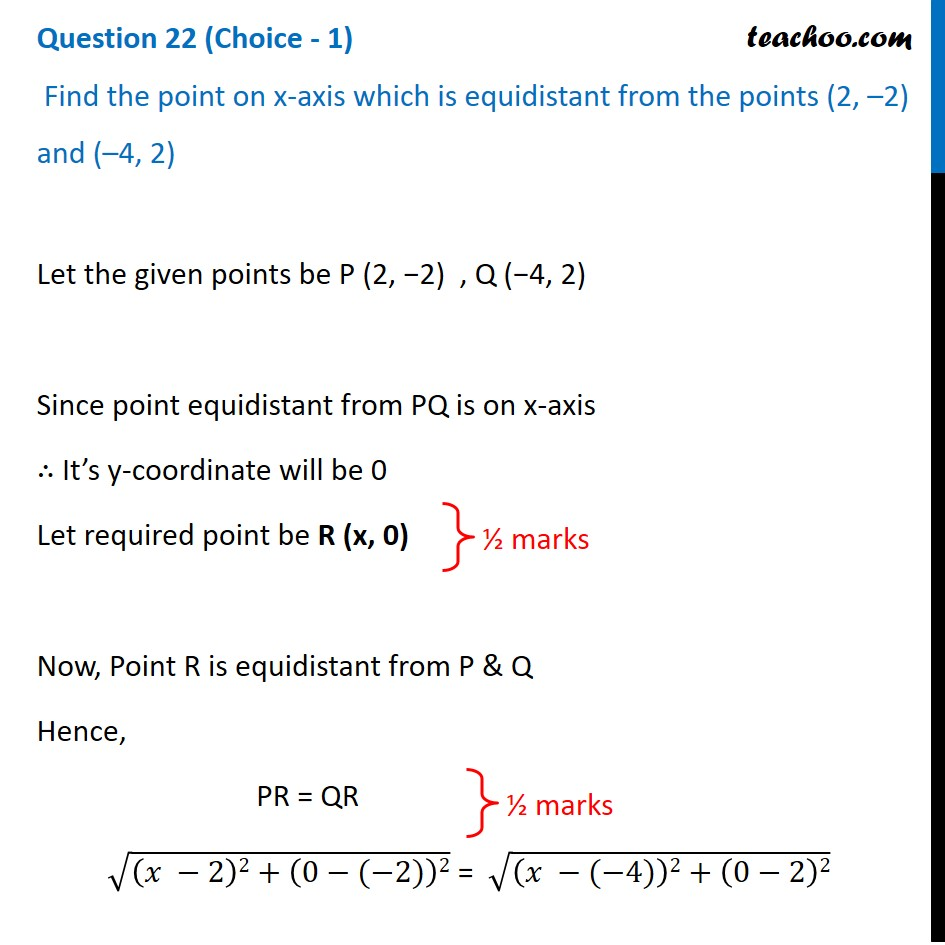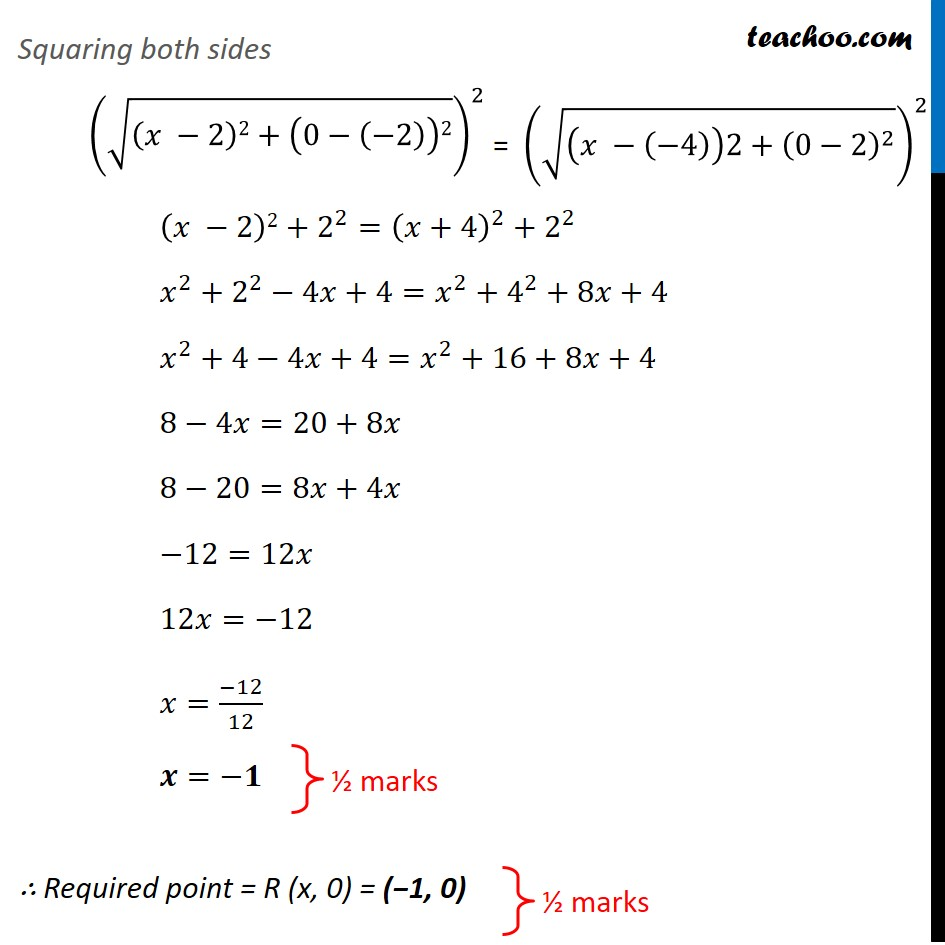## Find the point on x-axis which is equidistant from the points (2,–2) and (–4,2)1. Class 10
2. Solutions of Sample Papers for Class 10 Boards
3. CBSE Class 10 Sample Paper for 2021 Boards - Maths Standard

Transcript

Question 22 (Choice - 1) Find the point on x-axis which is equidistant from the points (2, –2) and (–4, 2) Let the given points be P (2, −2) , Q (−4, 2) Since point equidistant from PQ is on x-axis ∴ It’s y-coordinate will be 0 Let required point be R (x, 0) Now, Point R is equidistant from P & Q Hence, PR = QR √((𝑥 −2)2+(0−(−2))2) = √((𝑥 −(−4))2+(0−2)2) Squaring both sides (√((𝑥 −2)2+(0−(−2))2))^2 = (√((𝑥 −(−4))2+(0−2)^2 ))^2 (𝑥 −2)2+2^2=(𝑥+4)^2+2^2 𝑥^2+2^2−4𝑥+4=𝑥^2+4^2+8𝑥+4 𝑥^2+4−4𝑥+4=𝑥^2+16+8𝑥+4 8−4𝑥=20+8𝑥 8−20=8𝑥+4𝑥 −12=12𝑥 12𝑥=−12 𝑥=(−12)/12 𝒙=−𝟏 ∴ Required point = R (x, 0) = (−1, 0)

CBSE Class 10 Sample Paper for 2021 Boards - Maths Standard

Class 10
Solutions of Sample Papers for Class 10 Boards